`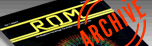ROM ISSUE 9 — DECEMBER/JANUARY 1985 / PAGE 32`

Lines Of Action
by Sol Guber

There are many action board games in which the possible moves are very dependent on the previous moves. The most famous of these is OTHELLO, where each move will change the configuration of the board, and a whole new strategy is needed. LINES OF ACTION by Claude Soucie is related to OTHELLO, but it is both more complicated, and simpler to play. Figure 1 shows the board at the beginning of play. The object of the game is to have your men touching one another vertically, horizontally, and diagonally. You can also take the other person, but if he has only one man left, he automatically wins (one man always touches itself). Also, the fewer men there are on the board, the easier it is to win.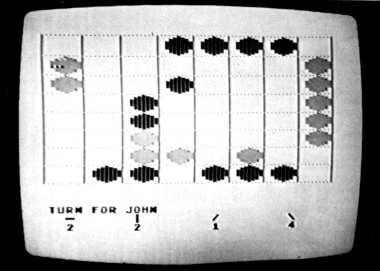The play of the game is simple. Each person, in turn, moves any man to another spot on the board. The direction that a man can move, and the number of squares that he can move, depends on his position on the board, and on the position of all the other men. A man can move horizontally, vertically, or diagonally the same number of squares as there are men in that direction. Let me explain this further. Looking at Figure 1 again, observe the WHITE man at B2. He can move horizontally, vertically, diagonally right, and diagonally left. In his horizontal row , there are six men. Thus, he can move (in theory) six squares to the left, or six squares to the right in a horizontal manner. There are two men in the vertical column, so he can move two squares up, or two squares down. There are two men in the diagonal left column, so he can move two squares in that direction. There are two men in the diagonal right, so he can move two squares in that direction. During play, you can see all the possible moves of a man, by moving the cursor to that man, and pressing the trigger. These are all possible moves. There are limits to the moves that can be made in the actual game. You cannot move off the board. You cannot move and land on your own man. You can jump over your own men, but you cannot jump over an opponent's man. You can land on your opponent, and he is removed from the board. Otherwise, the moves can be made in any manner.
Let me go into the way that the game is played. When the game is started, there is a question regarding instructions. A "Y" or "N" answer is expected. The computer asks for the names of the two players. The game can be played at two levels of difficulty. At the bottom of the screen, all the possible moves of any man can be determined by the Computer, and shown. If you do not want this to be done, answer the question with an "N." When it is a person's turn, his name will be shown at the bottom of the screen. White uses Joystick 1, and BLACK uses Joystick 2, to move a flashing cursor on the screen.
If the direction option is on, the cursor is moved to the man you wish to examine, and the trigger is pressed. The bottom display changes, and a marker is put on that man. The cursor is then moved to either the position to which you want to move that man, or to another man of the same color. Then the trigger is pressed. If it is to a man of the same color, the old marker is moved, and the information on the screen is updated. If it is not to a man of the same color, the move is checked to see if it is a legal one. If it is not. the move must be taken over. If it is a legal move, the man is moved to that square and removed from the old square. The board is checked to see if anyone has won, and then it is the other person's move. The game continues until someone wins.
The game has a large amount of complexity to it, yet it is very simple to learn. To help the beginner, there is the option to show on the bottom of the screen all the possible moves. For the advanced player, this is too distracting, and is not needed. The strategy of the game involves all the different men that can be moved. The decision whether to jump an opponent and remove him from the board is tempered with the idea that the fewer men on the board, the easier it is to win. Since the object of the game is the same for both players, each play must be both offensive and defensive at the same time.
To go through the working of the strategy, let's review the play of a game. In Figure 1, the men are set up as they would be at the start. The WHITE player is conservative, and plans his moves carefully. BLACK plays a more reckless game, and keeps trying to remove WHITE's men. The first move by WHITE is from A4 to C4. The man on A4 can move six squares in a horizontal position, and two squares in each of the vertical, the left diagonal, and the right diagonal positions. He has moved two squares downward in a vertical manner. On his next move, if he so wishes, he can move back to his original square. The BLACK man on C1 can move six squares vertically, three squares horizontally, and two squares either left diagonal, or right diagonal. He wishes to move three squares horizontally to the right, and lands on the WHITE man, removing him from the board in Figure 3. Again, this is a combination of plays. To be too aggressive, and to remove your opponent's men, will make it easier for him to win.
The play for the next few moves is rather straightforward. WHITE's next move is just a vertical one down. BLACK then moves horizontally from H3 to E3. White then moves vertically up from E8 to E5 in Figure 6. There are many more plays, and these are detailed in Table 1, which shows the whole game.
Let me skip ahead several moves to Figure 7, which corresponds to move 10 for WHITE. By Figure 7, Black has a decided advantage. He has ten of his eleven men touching, and all he needs is to move the man at H7 to the proper position, and he will win. White must prevent Black from bringing all his men together. To do this, White moves vertically up two to block BLACK's diagonal left move. If Figure 9, Black moves diagonally down from H7 to G8, so that on his next move, he can move diagonally left 3 up, and win the game. In Figure 10, White moves from G6 to F7 to block this move, since BLACK cannot jump over his opponent's men. Black moves from G8 to G6, to try some other method of winning the game.
WHITE now has a chance to make some counterattacks. In Figure 12, move 12, White goes from B 1 to D1. BLACK moves from G6 to G4, to win by a move to E2. WHITE moves from D6 to D3, on a vertically up move, removing the BLACK man on D3. White has now split up BLACK into two parts, and now BLACK has lost the offensive. In Figure 15, BLACK has moved from G4 to E2, to try to find some method of bringing his two groups together. WHITE moves from GI to G2, to try to take the man at E2. BLACK moves his man away from D2 to B2.
WHITE has changed the flow of the game. White now moves from G2 to E4, taking the BLACK man there. White is now trying to consolidate his men, in Figure 18. BLACK now moves from C2 to D2, and WHITE moves from D1 to El in move 16. In Figure 19, BLACK moves from A6 to C6, to try to get the man at E4. White counters by moving from F7 to C4, removing the BLACK man there. BLACK then moves from C6 to E4, taking WHITE. WHITE moves from F4 to F2. BLACK moves from E4 to F3, to try to take the man at F6, but WHITE does it first in Figure 26. BLACK moves from E5 to C3, taking WHITE there, and leaves himself open for WHITE's E1 to E3 move for the win.
I have gone through a game in a great deal of detail to show the flavor of it. It is very exciting, and contains portions of OTHELLO, checkers, and chess. The interesting part of the game is how each move changes the board for the other men. The major flaw is in Figure 27, where Black is so eager to move to C3 that he removes one man from that column, allowing WHITE a two move vertically, which removed the man. If the man were still at E5, then only a three vertical move would be allowed, and the man at E3 would have been safe.
I am willing to make cassette copies of this program for those that would like them. Please send a self addressed, stamped cassette mailer to me, plus \$3 to cover postage and handling.
Send the cassette to:

Sol Guber
717 Elkington
St. Louis, MO 63132
USA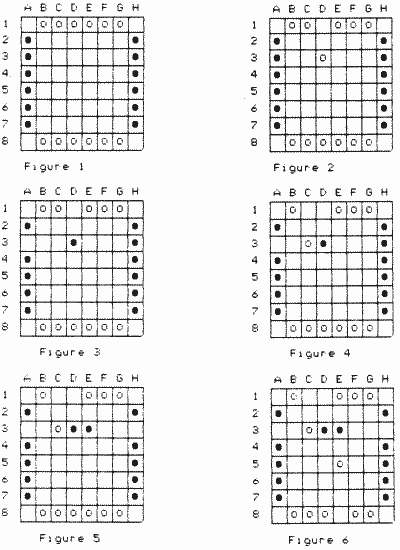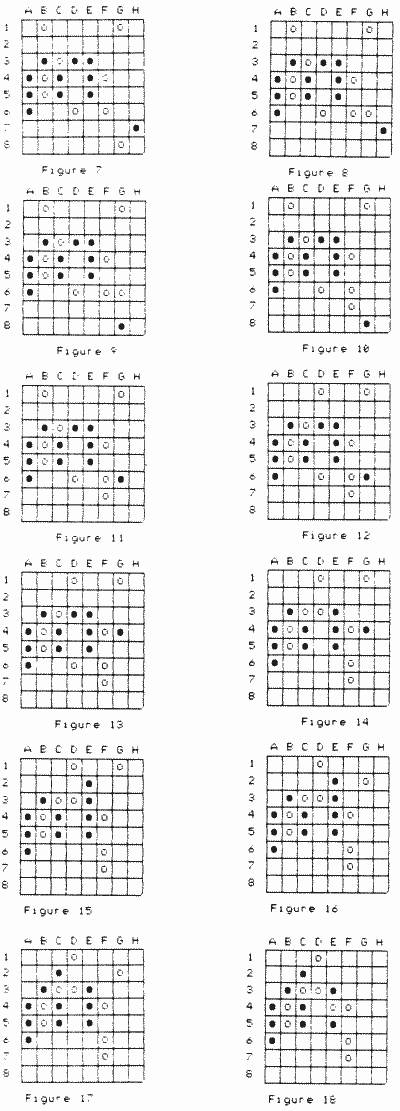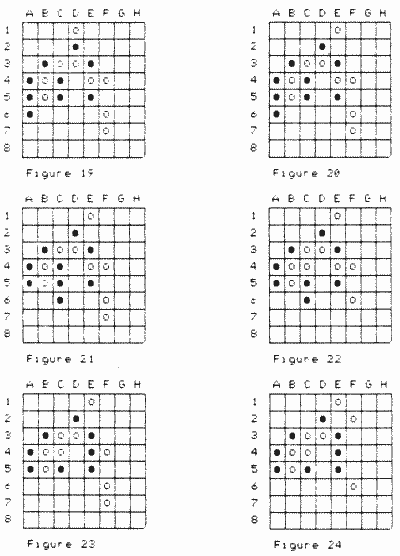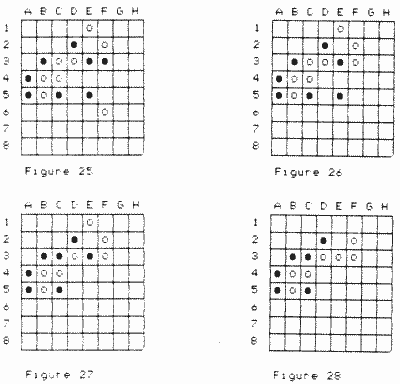TABLE ONE White Black 1. D1-D3 A3-D3 2 C1-C3 H3-E3 3. E8-E5 H2-E5 4 E1-B4 H4-E4 5. B8-B5 A2-B3 6 C8-C5 A4-B3 7. D8-D6 A7-A4 8. F8-F6 H6-F4 9. F1-F4 H5-C5 10. G8-G6 H7-G8 11. G6-F7 G8-G6 12. B1-D1 G6-G4 13. D6-D3 G4-E2 14. G1-G2 E2-C2 15. G2-E4 C2-D2 16. D1-E1 A6-C6 17. F7-C4 C6-E4 18. F4-F2 E4-F3 19. F6-C3 E5-C3 20. E1-E3 Underlined man is taken.

1 AGAIN=0::REM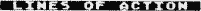BY SOL GUBER.
2 C1=1:C0=0:C2=2:C3=C2+C1:C4=C2+C2:C5=5:C6=C4+C2:C7=C5+C2:C8=C6+C2:C9=C8tC1:C10=C2+C8:C15=C10+C5:C14=C15-C1:C17=17
4 DIM R\$(120),S\$(120),PAT(C8):R\$(C1)=CHR\$(C0):R\$(120)=CHR\$(C0):R\$(C2)=R\$:C12=12:C11=C12-C1
5 DIM PLAYERS (20),A\$(C10),XDIF(C15),YDIF(C15):PLAYER\$(C1)=" ":PLAYER\$(20)=" ":PLAYER\$(C2)=PLAYER\$
6 IF AGAIN<>C0 THEN GO TO C10
7 GOSUS 4000:GOSUB 6000
8 TRAP C8:? "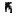D0 YOU NEED INSTRUCTIONS":INPUT A\$:IF A\$(1,1)="Y" THEN GOSUB 3000
10 GOSUB 3500:GRAPHICS C0:DIM MOVE(C4),MO(C7),KN(C2):GOSUB 1000
15 DX=CI2:DY=C3:DPX=DX:DPY=DY:PT=C2:POKE 752,C1:GOSUB 200
18 KN(C0)=12:KN(C1)=12:MOVE(C0)=C0
30 FOR I=C0 TO C1:FLG=C0:POKE 77,C0:VL=(I=C0)*C5+(I=C1)*C10
35 ? "TURN FOR ";PLAYER\$(C10*I+C1,C10*I+C10)
36 IF CFLAG=C1 THEN 45
38 IF MOVE(C0)=C0 THEN ? "                                ":GOTO 45
40 ? " -        |         /         \":? "  ";MOVE(C0),MOVE(C1),MOVE(C2),MOVE(C3)
45 COLOR C3:PLOT DPX+C3,DPY+C9:PLOT DPX+C4,DPY+C9:PLOT DPX+C3,DPY+C10:PLOT DPX+C4,DPY+C10
50 S=STICK(1):TR=STRIG(1):IF JOY=C1 INEN S=STICK(C0):TR=STRIG(C0)
53 IF TR=C0 AND FLG<>C1 THEN FLG=C1:KNT=(DPX+C6)/C9+C10*(DPY-C3)/C17:GOSUB 300:GOTO 35
55 IF TR=C0 AND FLG=C1 THEN 115
58 IF S=C15 THEN LOCATE DPX,DPY,Z:POKE 707,PEEK(704):Q=1^1^1:POKE 707,Z:Q=1^1^1:GOTO 50
60 DX=DPX+XDIF(S)*C9:DY=DPY+YDIF(S)*C17
63 IF DX<C3 OR RX>67 THEN 50
65 IF DY<C3 OR DV>122 THEN 50
85 LOCATE DPX+C6,DPY+C9,Z:COLOR Z:PLOT DPX+C3,DPY+C9:PLOT DPX+C4,DPY+C9:PLOT DPX+C3,DPY+C10:PLOT DPX+C4,DPY+C10
88 DPX=DX:DPY=DY
90 FOR Q=C1 TO 75:NEXT Q:GOTO 45
115 G0SUB 550:GOTO 320
120 SOUND C0,C0,C0,C0
135 ? "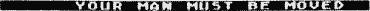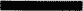":GOSUB 520:FOR WAIT=C1 TO 250:NEXT WAIT
140 FLG=C0: RETURN
195 NEXT I:GOTO 30
200 IF ASC(R\$(PT))=C0 THEN FOR Q=C0 TO C4:MOVE(Q)=C0:NEXT Q:RETURN
203 TPT=INT(PT/(C10):UPT=PT-TPT*C10
205 COUNT=CO:K3=TPT*10+C1:K4=TPT*C10+8:K5=C1:GOSUB 250:MOVE(C0)=COUNT
210 COUNT=C0:K3=UPT:K4=UPT+70:K5=C10:GOSUB 250:MOVE(C1)=COUNT
215 T1PT=TPT:U1PT=UPT:IF TPT+UPT>C9 THEN T1PT=C8-UPT:U1PT=C8-TPT
220 COUNT=C0:K3=PT-T1PT*C9:K4=PT+(U1PT-C1)*C9:K5=C9:GOSUB 250:MOVE(C2)=COUNT
225 T1PT=TPT:U1=T=UPT:IF UPT>TPT THEN U1PT=TPT+C1:T1PT=UPT-C1
230 COUNT=C0:K3=PT-(U1PT-C1)*C11:K4=PT+(C7-T1PT)*C11:K5=C11:GOSUB 250:MOVE(C3)=COUNT
235 RETURN
250 FOR K2=K3 TO K4 STEP K5:IF ASC(R\$(K2))<>C0 THEN COUNT=C(    )UNT+C1
260 NEXT K2
270 RETURN
380 GOSUB 500
302 IF I=C0 AND ASC(R\$(KNT))<>C5 THEN 135
3O3 IF Z=C1 AND ASC(R\$(KNT))<>C10 THEN 135
385 PT=(DPX+C6)/C9+C10*(DPY-C3)/C17:GOTO 200
320 K2=(DPX+C6)/C9+C10*(DPY-C3)/C17:IF ASC(R\$(K2))=VL THEN FLG=C0:GOSUB 600:KNT=K2:GOSUB 305:GOSUB 503:GOTO 35
322 IF ABS(K2-KNT)=MOVE(C0) THEN 340
324 IF ABS(K2-KNT)=MOVE(C1)*C10 THEN 340
326 IF ABS(K2-KNT)=MOVE(C2)*C9 THEN 340
328 IF ABS(K2-KNT)=MOVE(C3)*C11 THEN 340
338 ? "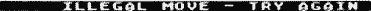":GOSUB 520:GOSUB 600:FLG=C0:FOR WAIT=C1 TO 250:NEXT WAIT:GOTO 35
340 VL=(I=C0)*5+(I=C1)*10:K4=K2-KNT
342 IF K4/C11=INT(K4/C11) THEN K5=11:GOTO 350
344 IF K4/C10=INT(K4/C10) THEN K5=C10:GOTO 350
346 IF K4/C9=INT(K4/C9) THEN K5=C9:GOTO 350
348 K5=C1
350 IF K2<KNT THEN K5=-KS
353 FOR Q=KNT TO K2-K5 STEP K5:IF ASC(R\$(Q))<>VL AND ASC(R\$(Q))<>C0 THEN 390
355 NEXT Q
358 IF ASC(R\$(K2))=VL THEN ? "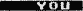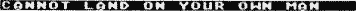":GOSUB 520:FLG=C0:GOSUB 600:GOTO 31
360 IF ASC(R\$(K2))<>C0 THEN KN((I=C0))=KN((I=C0))-C1
363 COLOR (I=C0)*C7+(I=C1)*C5:P=INT(K2/C10):X=(K2-P*C10)*C9-C6:Y=P*C17+C3:GOSUB 2300
365 COLOR C0:P=INT(KNT/C10):X=(KNT-P4*C10)*C9-C6:Y=P*C17+C3:GOSUB 2300
368 R\$(KHT,KNT)=CHR\$(C0):R\$(K2,K2)=CHR\$(VL)
370 M1=C0:VL=C5:GOSUB 400:M1=C1:VL=C10:GOSUB 400:MOVE(C0)=C0:GOTO 195
390 ? "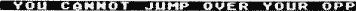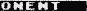":GOSUB 520:GOSUB 600:FLG=C0:FOR WAIT=C1 TO 250:NEXT WAIT:GOTO 35
400 J=C1:S\$=R\$:COUNT=C1
403 IF ASC(S\$(J))<>VL THEN J=J+1:GOTO 403
405 K=C0
412 P1=J+MOVT(K):ZF P1<C1 THEN 450
415 K2=ASC(S\$(P1)):IF K2<>VL THEN 450
420 S\$(J,J)=CHR\$(VL+K*16):COUNT=COUNT+C1:J=P1:GOTO 405
450 K=K+C1:IF K<C8 THEN 412
455 S\$(J,J)=CHR\$(VL+112):IF COUNT>=KN(M1) THEN GOTO 700+100*M1
460 FOR K=C0 TO C7:P1=J+MOVT(K):IF P1<C1 THEN 480
463 K2=ASC(S\$(P1)):IF K2=C0 THEN 480
465 IF K2=VL+112 THEN 480
468 IF K2<C11 THEN 480
470 K=INT(K2/16)+1:J=P1:GOTO 412
480 NEXT K:RETURN
500 SOUND 0,75,18,12:FOR I1=1 TO 188:NEXT I1:SOUND 0,0,0,0
503 IF ASC(R\$(KNT))<>VL THEN FLG=C0:RETURN
510 COLOR 1:PLOT DPX+C4,DPY+C3:PLOT DPX+C5,DPY+C3:RETURN
520 SOUND 0,75,12,12:FOR I1=1 TO 100:NEXT I1:SOUND 0,0,0,0:RETURN
550 SOUND 0,125,10,12:FOR I1=1 TO 100:NEXT I1:SOUND 0,0,0,0:RETURN
580 SOUND 0,125,12,12:FOR I1=1 TO 100:NEXT I1:SOUND 0,0,0,0:RETURN
600 P=INT(KNT/C10):X=(KNT-P*C10)*C9-C6:Y=P*C17+C3
603 LOCATE X+C4,Y+C9,Z:COLOR Z
610 PLOT X+C4,Y+C3:PLOT X+C5,Y+C3:RETURN
700 ? "";PLAYER\$(C1,C10):? "IS THE WINNER":FOR I1=0 TO 255 STEP 0.25:POKE 788,I1:NEXT I1
710 GOTO 805
800 ? "";PLAYER\$(11,20):? "IS THE WINNER":FOR I1=0 TO 255 STEP 0.5:POKE 708,I1:NEXT I1
805 FOR WAIT=C1 TO 300:NEHT WAIT:POKE 764,255
810 TRAP 810:? "PLAY AGAIN";:INPUT PLAYER\$:IF PLAVER\$(1,1)="Y" THEN CLR :AGAIN=1:TRAP 40000:GOTO 2
820 TRAP 40000:GRAPHXCS 0:END
1000 GOSUB 4000:GO5UB 4045
1005 RESTORE 1020
1010 FOR I=C0 TO C7:READ A:MOVT(I)=A:NEXT I
1020 DATA -11,-1,9,10,11,1,-9,-10
1200 ? "WHITE PLAYER, PLEASE ENTER YOUP NAME":INPUT A\$:IF LEN(A\$)=C0 THEN 1200
1205 PLAYER\$(1,LEN(A\$))=A\$:I=C0
1210 ? "BLACK PLAYER, PLEASE ENTER YOUR NAME":INPUT A\$:IF LEN(A\$)=C0 THEN 1200
1215 PLAYER\$(11,10+LEN(A\$))=A\$:I=C1
1220 RESTORE 1225
1225 DATA 1,1,1,-1,1,0,0,0,-1,1,-1,-1,-1,0,0,0,0,1,0,-1,0,0
1230 FOR Q=C5 TO 15:READ X,Y:XDIF(Q)=X:YDIF(Q)=Y:NEXT Q
1250 ? "ADVANCED PLAY (Y,N)";:INPUT A\$:IF LEN(A\$)=C0 THEN RETURN
1260 IF A\$(1,1)="Y" THEN CFLAG=C1
1300 RETURN
2300 PLOT X+C2,Y+C5:DRAWTO X+C2,Y+C11
2310 PLOT X+C3,Y+C3:DRAWTO X+C3,Y+C14
2320 PLOT X+C4,Y+C2:DRAWTO X+C4,Y+C15
2340 PLOT X+C5,Y+C1:DRAWTO X+C5,Y+16
2350 PLOT X+C6,Y+C2:DRAWTO X+C6,Y+C15
2360 PLOT X+C7,Y+C3:DRAWTO X+C7,Y+C14
2370 PLOT X+C8,Y+C5:DRAWTO X+C8,Y+C11
2380 RETURN
3000 TRAP 40000:GRAPHICS C0:POKE 709,C0:POKE 710,190:POKE 712,190:POKE 752,C1
3002 ? "":? :?
3005 ? "THIS IS A VERY INTRICATE GAME. THE    OBJECT IS TO POSITION ALL YOUR PIECES"
3030 ? "IN SUCH A MANNER THAT THEY TOUCH ONE  ANOTHER, REGARDLESS OF THE ACTUAL"
3015 ? "FORMATION OR THE NUMBER OF MEN LEFT   ON THE BOARD."
3020 ? "THINK OF YOUR MEN AS PIECES OF A      CHAIN. YOUR JOB IS TO CONNECT ALL"
3025 ? "THE LINKS.  THE LENGTH OR SHAPE OF    THE CHAIN IS IRRELEVANT. IN FACT,"
3028 ? "THE MORE LINKS YOU HAVE, THE MORE     DIFFICULT YOUR JOB BECOMES."
3029 ? "FOR EXAMPLE, IF YOU ONLY HAVE ONE     PIECE LEFT, YOU AUTOMATICALLY WIN!"
3030 ? "YOUR MOVEMENTS ARE GOVERNED BY        CERTAIN RULES."
3050 POKE 764,255:POSITION 6,21:? "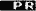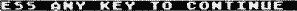"
3060 IF PEEK(764)=255 THEN 3060
3065 ? "":? :?
3070 ? "ALTHOUGH YOU MAY MOVE VERTICALLY,     HORIZONTALLY, DIAGONALLY, FORWARD"
3080 ? "AND IN REVERSE, YOUR LIMITS ARE AS    FOLLOWS:"
3085 ? "  1. THE NUMBER OF SQUARES YOUR PIECE CAN MOVE IS DETERMINED BY ITS PRESENT"
3090 ? "POSITION, AS WELL AS BY HOW MANY OTHERMEN ARE IN THE SAME ROW OR COLUMN."
3092 ? "AFTER YOU INDICATE WHICH PIECE YOU    INTEND TO PLAY WITH, THE COMPUTER WILL"
3094 ? "↑DISPLAY ITS VARIOUS AVAILABLE MOVES."
3095 ? "  2. YOU MAY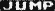OVER YOUR MEN, BUT NOT OVER YOUR OPPONENT'S."
3100 ? "  3. YOU ARE ALLOWED TO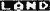ON YOUR OPPONENT'S PIECE (NOT YOURS). THIS    ELIMINATES THAT PIECE";
3105 ? " FROM THE GAME."
3110 POKE 764,255:POSITION 6,21:? ""
3115 IF PEEK(764)=255 THEN 3115
3120 ? "":? :?
3125 ? "IN THE ADVANCED-PLAY MODE, THE ALLOW-"
3128 ? "ABLE MOVES FOR THE VARIOUS PIECES     WILL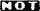BE SHOWN."
3130 ? "POSITION THE CURSOR ABOVE. THE MAN    YOU WISH TO MOVE AND PRESS THE FIRE-"
3135 ? "BUTTON.  THEN MOVE THE CURSOR TO THE  SQUARE WHICH YOU WANT TO LAND ON AND"
3140 ? "PRESS THE FIREBUTTON AGAIN.  IF YOU   CHANGE YOUR MIND, SIMPLY LAND ON"
3142 ? "THE SAME MAN YOU STARTED OUT WITH.    YOU MAY THEN MAKE A DIFFERENT MOVE."
3144 ? "IF YOUR MOVE IS ILLEGAL, THE COMPUTER WILL GIVE YOU THE REASON WHY."
3145 ? "IN A TWO-JOYSTICK GAME, WHITE ALWAYS  USES PORT #l; BLACK USES PORT #2."
3148 ? "THINK AHEAD BEFORE MOVING. GOOD LUCK!"
3150 POKE 764,255:POSITION 6,21:? ""
3160 IF PEEK(764)=255 THEN 3160
3400 POKE 752,C0:RETURN
3500 TRAP 3500:? "":POSITION 2,12:? "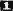OR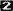JOYSTICKS";:INPUT JOY:IF JOY<C1 OR JOY>C2 THEN 3500
3510 TRAP 40000:RETURN
4000 RESTORE 4005:GRAPHICS 8:FOR I=704 TO 712:READ A:POKE I,A:NEXT I
4005 DATA 12,76,86,68,130,0,190,14,190
4010 Z=PEEK(560)+256*PEEK(561):POKE 559,0:POKE Z+166,143:POKE 513,6:POKE 512,0
4015 RESTORE 4030
4020 FOR I=1536 TO 1546:READ A:POKE I,A:NEXT I
4030 DATA 72,169,0,141,10,212,141,27,208,104,64
4040 POKE 54286,192:POKE 559,34
4042 POKE 87,C10:POKE 623,128:RETURN
4045 COLOR C4
4050 FOR I=C3 TO 139 STEP C17:PLOT C3,I:DRAWTO 75,I:NEXT I
4060 FOR I=C3 TO 75 STEP C9:PLOT I,C3:DRAWTO I,139:NEXT I
4070 Y=C3:COLOR C7
4073 FOR X=C12 TO 60 STEP C9:GOSUB 2300:NEXT X
4075 Y=122:FOR X=C12 TO 60 STEP C9:GOSUB 2300:NEXT X
4080 COLOR C5:X=C3:FOR Y=20 TO 105 STEP C17:GOSUB 2300:NEXT Y
4090 X=66:FOR Y=20 TO 105 STEP C17:GOSUB 2300:NEHT Y
4100 FOR X=C2 TO C7:R\$(X,X)=CHR\$(C5):NEXT X
4110 FOR X=72 TO 77:R\$(X,X)=CHR\$(C5):NEXT X
4120 FOR X=C11 TO 61 STEP 10:R\$(X,X)=CHR\$(C10):NEXT X
4130 FOR X=18 TO 68 STEP 10:R\$(X,X)=CHR\$(C10):NEXT X
4300 RETURN
6000 RESTORE 6100
6002 COLOR C4:FOR I=C3 TO 139 STEP 17:PLOT C3,I:DRAWTO 75,I:NEXT I
6004 FOR I=C3 TO 75 STEP 9:PLOT X,3:DRAWTO I,139:NEXT I
6010 GOSUB 6500
6030 FOR P=C1 TO 40:X=PEEK(704)
6048 FOR I=704 TO 711:POKE I,PEEK(I+1):NEXT I:POKE 711,X:NEXT P
6045 SOUND C0,C0,C0,C0
6050 RETURN
6100 DATA 22,44,3,33,41,3,44,46,3,55,37,3,66,51,3,35,47,7,36,38,7
6110 DATA 6,33,1,15,35,1,24,52,1,42,47,1,51,46,1,62,51,4,63,47,4,64,44,4
6115 DATA 71,39,5,72,53,5,73,34,5,74,37,5,75,50,5
6500 FOR P=1 TO 20:READ Z,K,K2:COLOR K
6520 TR=57344+C8*K+C1:FOR J=C0 TO C7:PAT(J)=PEEK(TR+J):NEXT J
6600 X4=INT (Z/C10):Y4=Z-X4*C10:X4=X4*17+C6:Y4=Y4*C9-C6
6610 FOR J=C0 TO C6:K2=PAT(J):SOUND C0,K2,C10,C10
6620 FOR J2=C0 TO CS
6630 IF K2/C2<>INT(K2/C2) THEN PLOT Y4+C8-J2,X4+J*C2:PLOT Y4+C8-J2,X4+J*C2+C1
6640 K2=INT(K2/C2):NEXT J2
6660 NEXT J:NEXT P:RETURN